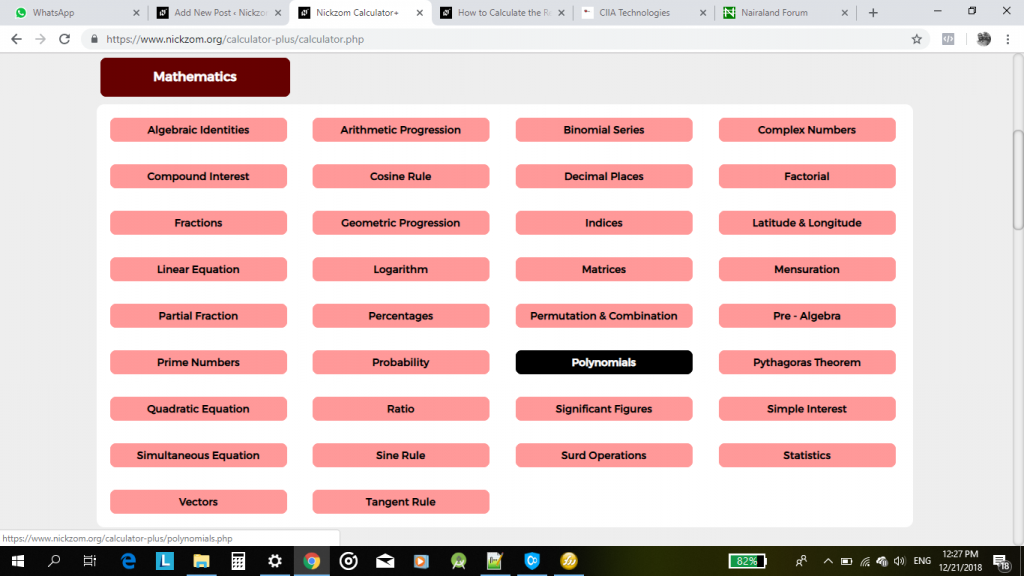## Nickzom Calculator Calculates and Solves for Polynomials | Cubic and Quartic

According to Wikipedia,

In mathematics, a polynomial is an expression consisting of variables (also called indeterminates) and coefficients, that involves only the operations of additionsubtractionmultiplication, and non-negative integer exponents of variables.

Nickzom Calculator is the very first calculator encyclopedia to come out from Nigeria. It is capable of solving over 50,000 calculations and presenting the steps (workings). Fortunately, Nickzom Calculator can compute conception and best intercourse dates.

You can access Nickzom Calculator via any of these means:
Web – https://www.nickzom.org/calculator-plus
Android (Paid) – https://play.google.com/store/apps/details?id=org.nickzom.nickzomcalculator
Android (Free) – https://play.google.com/store/apps/details?id=com.nickzom.nickzomcalculator
Apple (Paid) – https://itunes.apple.com/us/app/nickzom-calculator/id1331162702?mt=8

Once, you have access to Nickzom Calculator proceed to the Calculator Map, click on Polynomials under Mathematics sectionFor Nickzom Calculator, there are two polynomials which are cubic and quartic.

A cubic equation or polynomial has a degree or order of 3 whereas a quartic equation or polynomial has a degree or order of 4.Let’s start with a cubic equation